### Restrictor, Long Orifice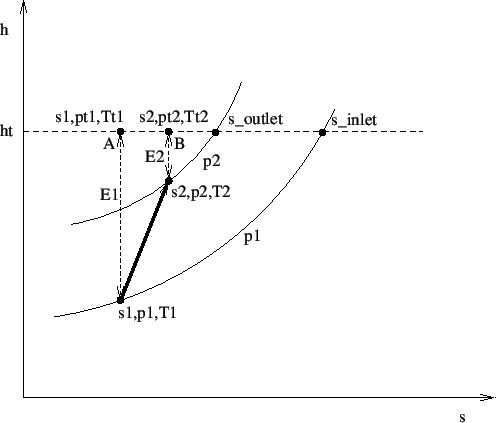Properties: adiabatic, not isentropic, symmetric,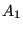inlet based restrictor

Restrictors are discontinuous geometry changes in gas pipes. The loss factor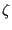can be defined based on the inlet conditions or the outlet conditions. Focusing on the h-s-diagram (entalpy vs. entropy) Figure (92), the inlet conditions are denoted by the subscript 1, the outlet conditions by the subscript 2. The entropy loss from state 1 to state 2 is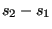. The process is assumed to be adiabatic, i.e.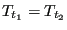, and the same relationship applies to the total entalpy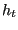, denoted by a dashed line in the Figure.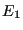denotes the kinetic energy part of the entalpy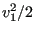, the same applies to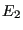. Now, the loss coefficientbased on the inlet conditions is defined by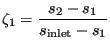(118)

and based on the outlet conditions by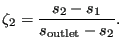(119)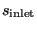is the entropy for zero velocity and isobaric conditions at the inlet, a similar definition applies to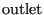. So, for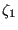the increase in entropy is compared with the maximum entropy increase from state 1 at isobaric conditions. Now we have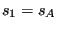and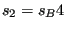consequently,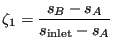(120)

and based on the outlet conditions by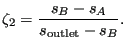(121)

Using Equation (51) one obtains:(122)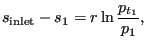(123)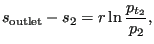(124)

from which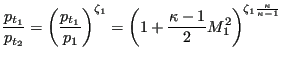(125)

ifis defined with reference to the first section (e.g. for an enlargement, a bend or an exit) and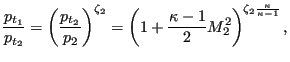(126)

ifis defined with reference to the second section (e.g. for a contraction).

Using the general gas equation (37) finally leads to (for):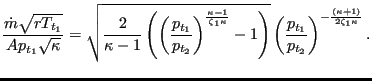(127)

This equation reaches critical conditions (choking,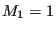) for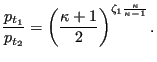(128)

Similar considerations apply to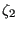.

Restrictors can be applied to incompressible fluids as well by specifying the parameter LIQUID on the *FLUID SECTION card. In that case the pressure losses amount to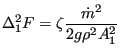(129)

and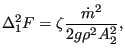(130)

respectively.

A long orifice is a substantial reduction of the cross section of the pipe over a significant distance (Figure 93).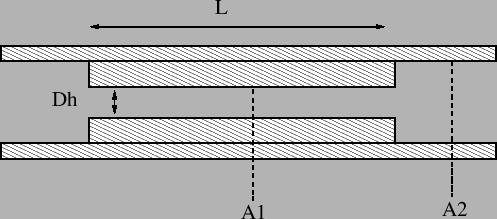There are two types: TYPE=RESTRICTOR LONG ORIFICE IDELCHIK with loss coefficients according to  and TYPE=RESTRICTOR LONG ORIFICE LICHTAROWICZ with coefficients taken from . In both cases the long orifice is described by the following constants (to be specified in that order on the line beneath the *FLUID SECTION, TYPE=RESTRICTOR LONG ORIFICE IDELCHIK or TYPE=RESTRICTOR LONG ORIFICE LICHTAROWICZ card):

• reduced cross section.
• full cross section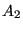.
• hydraulic diameter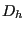defined by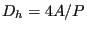where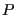is the perimeter of the cross section.
• Length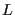of the orifice.
• oil mass flow in the restrictor (only if the OIL parameter is used to define the kind of oil in the *FLUID SECTION card)
• not used (internally: oil material number)

A restrictor of type long orifice MUST be preceded by a restrictor of type user with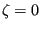. This accounts for the reduction of cross section fromto.

By specifying the parameter LIQUID on the *FLUID SECTION card the loss is calculated for liquids. In the absence of this parameter, compressible losses are calculated.

Example files: restrictor, restrictor-oil.